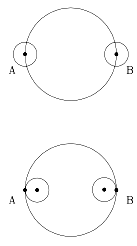# (8c) The Moon's Distance--1Index5c. Coordinates6. The Calendar6a. Jewish Calendar7.Precession8. The Round Earth8a. The Horizon8b. Parallax8c. Moon dist. (1)8d. Moon dist. (2)9a. Earth orbits Sun?9c. Copernicus         to Galileo10. Kepler's Laws10a. Scale of Solar Sys.11a. Ellipses         and First Law Aristarchus around 270 BC derived the Moon's distance from the duration of a lunar eclipse (Hipparchus later found an independent method).     It was commonly accepted in those days that the Earth was a sphere (although its size was only calculated a few years later, by Eratosthenes ). Astronomers also believed that the Earth was the center of the universe, and that Sun, Moon, planets and stars all orbited around it. It was only natural, then, that Aristarchus assumed that the Moon moved in a large circle around Earth.     Let R be the radius of that circle and T the time it takes the Moon to go around once, about one month. In that time the Moon covers a distance of 2 πR, where π~ 3.1415926... (pronounced "pi") is a mathematical constant, the ratio (circumference/diameter) in a circle.     An eclipse of the Moon occurs when the Moon passes through the shadow of the Earth, on the opposite side from the Sun (therefore, it must be a full Moon). If r is the radius of the Earth, the shadow's width is close to 2r. Let t be the time it takes the mid-point of the Moon to cross the center of the shadow, about 3 hours (in eclipses of the longest duration, when the Moon crosses the center of the shadow).     If the Moon moves around Earth at a constant speed--and it takes time T to cover 2π R ~ 6.28R, and time t to cover 2r--then 6.28 R / 2 r     =     T/t From this Aristarchus obtained R/r     ~     60 which fits the average distance of the Moon accepted today, 60 Earth radii.

### A Few Extra Details

The word "about" was used here more than once. For instance, the orbital period of the Moon was stated to be "about" one month. In fact, the length of the "lunar month" from one new Moon to the next (or from one full Moon to the next) is 29.53 days, but the Moon's orbital period is actually 2.21 days shorter (this is discussed in the section on the calendar).

Viewed from Earth, a "new Moon" (occuring between the time a thin crescent is last seen before sunrise and the time one is seen shortly after sunset) happens when the Moon in its apparent motion around the sky overtakes the Sun. However, by the time of the next new Moon, the Sun's position in the sky has already shifted. If the Sun takes 12 months to go around the sky (or around the ecliptic, or around the zodiac), then in one month it completes 1/12 of its circuit. The Moon must therefore complete [1+(1/12)] circuits to catch up with the Sun again, and the lunar month ("synodic period") is about 1/12 of a month longer than the actual period of 27.32 days.Also, the Earth's shadow has only approximately the width of 2r. It would have very nearly a width of 2r if the Sun were a point-like light source (exactly that width if it were infinitely far away). Actually, however, the Sun is large enough to appear as a disk which covers about half a degree of the sky. As a result, the Earth's shadow is not a cylinder but a gradually narrowing cone, and at the Moon's distance it is already about 25% narrower than 2r.

Here is another way of looking at the same process. Suppose we observe the eclipse from the Moon. Seen from there, the Earth moves from east to west--from A to B in the drawing, assuming the eclipse is of greatest length (i.e. the middle of the Earth passes in front of the Sun).

The eclipse begins when the last bit of the western edge of the Sun passes point A (bottom of the drawing shown) and ends when the first bit of the eastern edge of the Sun pokes out at point B. That takes less time than it takes for the center of the Sun to pass from A to B (top drawing) which would be the duration of the eclipse if the Sun were a tiny point source, located at its middle.

Teachers using this web page will find a related lesson plan at Lhipprc2.htm
It belongs to a set of lesson plans whose home page is at Lintro.htm.

Further Exploring:    #8d  How distant is the Moon?--2

Author and Curator:   Dr. David P. Stern
Mail to Dr.Stern:   stargaze("at" symbol)phy6.org .

Last updated: 9-18-2004